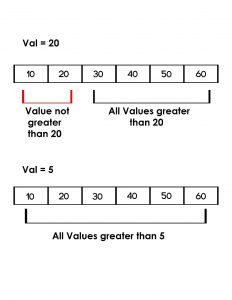# Python | Check if all the values in a list that are greater than a given value

Given a list, print all the values in a list that are greater than the given valueExamples:

```Input : list = [10, 20, 30, 40, 50]
given value = 20
Output : No

Input : list = [10, 20, 30, 40, 50]
given value = 5
Output : Yes
```

## Recommended: Please try your approach on {IDE} first, before moving on to the solution.

Method 1: Traversal of list

By traversing in the list, we can compare every element and check if all the elements in the given list are greater than the given value or not.

 `# python program to check if all  ` `# values in the list are greater  ` `# than val using traversal ` ` `  `def` `check(list1, val): ` `     `  `    ``# traverse in the list ` `    ``for` `x ``in` `list1: ` ` `  `        ``# compare with all the values ` `        ``# with val ` `        ``if` `val>``=` `x: ` `            ``return` `False`  `    ``return` `True` `     `  `     `  `# driver code  ` `list1 ``=``[``10``, ``20``, ``30``, ``40``, ``50``, ``60``] ` `val ``=` `5` `if``(check(list1, val)): ` `    ``print``"Yes"` `else``: ` `    ``print``"No"` ` `  `val ``=` `20`  `if``(check(list1, val)): ` `    ``print``"Yes"` `else``: ` `    ``print``"No"`

Output:

```Yes
No
```

Method 2: Using all() function:

Using all() function we can check if all values are greater than any given value in a single line. It returns true if the given condition inside the all() function is true for all values, else it returns false.

 `# python program to check if all  ` `# values in the list are greater ` `# than val using all() function ` ` `  `def` `check(list1, val): ` `    ``return``(``all``(x > val ``for` `x ``in` `list1)) ` `     `  `     `  `# driver code  ` `list1 ``=``[``10``, ``20``, ``30``, ``40``, ``50``, ``60``] ` `val ``=` `5` `if``(check(list1, val)): ` `    ``print``"Yes"` `else``: ` `    ``print``"No"` ` `  `val ``=` `20`  `if` `(check(list1, val)): ` `    ``print``"Yes"` `else``: ` `    ``print``"No"`

Output:

```Yes
No
```

My Personal Notes arrow_drop_upStriver(underscore)79 at Codechef and codeforces D

If you like GeeksforGeeks and would like to contribute, you can also write an article using contribute.geeksforgeeks.org or mail your article to contribute@geeksforgeeks.org. See your article appearing on the GeeksforGeeks main page and help other Geeks.

Please Improve this article if you find anything incorrect by clicking on the "Improve Article" button below.

Article Tags :
Practice Tags :

Be the First to upvote.

Please write to us at contribute@geeksforgeeks.org to report any issue with the above content.